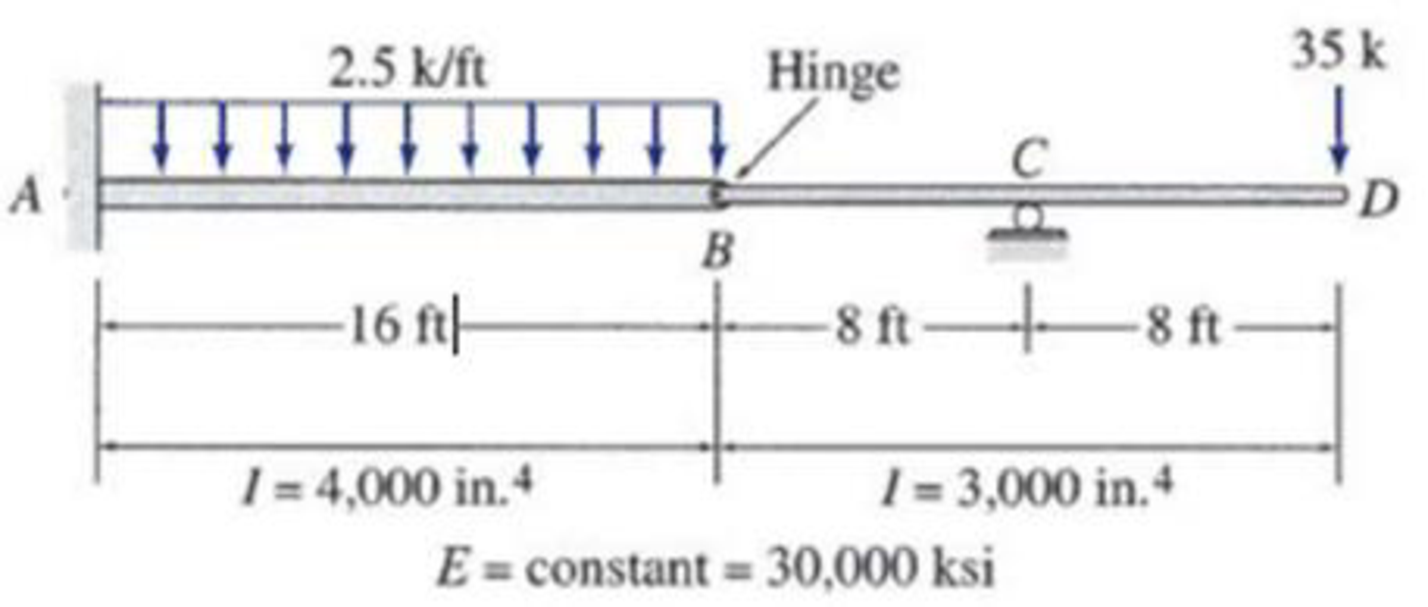# 6.33 and 6.34 Use the moment-area method to determine the slopes and deflections at point B and D of the beam shown FIG.P6.34, P6.60

#### Solutions

Chapter
Section
Chapter 6, Problem 34P
Textbook Problem
117 views

## 6.33 and 6.34 Use the moment-area method to determine the slopes and deflections at point B and D of the beam shownFIG.P6.34, P6.60

To determine

Find the slope θB&θD and deflection ΔB&ΔD at point B and D of the given beam using the moment area method.

### Explanation of Solution

Given information:

The Young’s modulus (E) is 30,000 ksi.

The moment of inertia of the section AB is (I) is 4,000in.4.

The moment of inertia of the section BD is (I) is 3,000in.4.

Calculation:

Consider Young’s modulus (E) of the beam is constant.

To draw a M/EI diagram, the reactions are calculated by considering all the loads acting in the beam. The calculation of positive moment at point A is calculated by simply considering the point load of 35 kips and the reactions calculated by considering all the loads acting in the beam.

Show the free body diagram of the given beam as in Figure (1).

Refer Figure (1),

Consider upward is positive and downward is negative.

Consider clockwise is negative and counterclowise is positive.

Refer Figure (1),

Consider reaction at A and C as RA and RC.

Determine the reaction at C;

RC×(8)(35×16)=0RC=5608RC=70kips

Determine the reaction at support A;

V=0RA+RC(2.5×16)35=0RA=7570RA=5kips

Determine the moment at A:

MA=(35×32)+(70×24)(25×16×162)=240kips-ft=240kips-ft(Clockwise)

Show the reactions of the given beam as in Figure (2).

Determine the bending moment at B;

MB=70×835×16=560560=0

Determine the bending moment at C;

MC=(35×8)=280kips-ft

Determine the bending moment at D;

MD=(5×32)(70×8)240+(2.5×16×(162+16))=720+240+960=0

Determine the positive bending moment at A;

MA=(35×32)+70×24=1,120+1,680=560kips-ft

Show the reaction and point load of the beam as in Figure (3).

Determine the value of M/EI;

MEI=560kips-ftEI

Substitute 4I3 for I.

MEI=560kips-ftE(4I3)=1,680kips-ft4EI=420kips-ftEI

Show the M/EI diagram of the beam as in Figure (4).

Show the elastic curve as in Figure (5).

The slope at point B can be calculated by evaluating the change in slope between A and B.

Express the change in slope using the first moment-area theorem as follows:

θB(left)=θAB=AreaoftheM/EIbetweenAandB=Areaoftriangle+Areaofparabola=[12×b1×h113×b2×h2]

Here, b is the width and h is the height of the respective triangle and rectangle.

Substitute 16 ft for b1, 420EI for h1, 16 ft for b2, and 240EI for h2.

θB(left)=[12×16×420EI13×16×240EI]=2,080kips-ft2EI

Determine the slope B (left) using the relation;

θB(left)=2,080kips-ft2EI

Substitute 30,000 ksi for E and 4,000in.4 for I.

Hence, the slope at B (left) is 0.0033rad_.

Determine the deflection at B using the relation;

Substitute 420EI for MEI, (12×16×(23×16)) for area of triangle, (240EI) for MEI, and (13×16×(34×16)) for area of triangle.

ΔBA=[420EI(12×16×(23×16))+(240EI)(13×16×(34×16))]=1EI(35,84015,360)=20,480kips-ft3EI

Determine the deflection at B (left) using the relation;

ΔBA=20,480kips-ft3EI

Substitute 30,000 ksi for E and 3,000in.4 for I.

ΔBA=20,480kips-ft3×(12in.1ft)330,0000×3,000=0.39in.()

Hence, the deflection at B (left) is 0.39in.()_.

Determine the deflection between B and C using the relation;

Here, b is the width and h is the height of the triangle

### Still sussing out bartleby?

Check out a sample textbook solution.

See a sample solution

#### The Solution to Your Study Problems

Bartleby provides explanations to thousands of textbook problems written by our experts, many with advanced degrees!

Get Started

Find more solutions based on key concepts
Calculate the thermal resistance due to convection for the following situations: a. warm water with h = 200 W/m...

Engineering Fundamentals: An Introduction to Engineering (MindTap Course List)

Principles of Information Systems (MindTap Course List)

What two line types work together to show sizes on an engineering drawing?

Precision Machining Technology (MindTap Course List)

Low Battery Life You have had your laptop for more than one year and notice that your battery is losing its cha...

Enhanced Discovering Computers 2017 (Shelly Cashman Series) (MindTap Course List)

Describe the strategy of defense.

Management Of Information Security

If your motherboard supports ECC DDR3 memory, can you substitute non-ECC DDR3 memory?

A+ Guide to Hardware (Standalone Book) (MindTap Course List)

What do the two pressure gauges on a regulator show?

Welding: Principles and Applications (MindTap Course List)